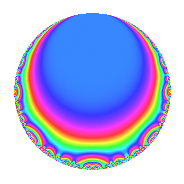# Properties

 Label 8008.2.a.gLevel 8008 Weight 2 Character orbit 8008.a Self dual Yes Analytic conductor 63.944 Analytic rank 1 Dimension 2 CM No Inner twists 1

# Related objects

## Newspace parameters

 Level: $$N$$ = $$8008 = 2^{3} \cdot 7 \cdot 11 \cdot 13$$ Weight: $$k$$ = $$2$$ Character orbit: $$[\chi]$$ = 8008.a (trivial)

## Newform invariants

 Self dual: Yes Analytic conductor: $$63.9442019386$$ Analytic rank: $$1$$ Dimension: $$2$$ Coefficient field: $$\Q(\sqrt{5})$$ Coefficient ring: $$\Z[a_1, a_2, a_3]$$ Coefficient ring index: $$1$$ Fricke sign: $$1$$ Sato-Tate group: $\mathrm{SU}(2)$

## $q$-expansion

Coefficients of the $$q$$-expansion are expressed in terms of $$\beta = \frac{1}{2}(1 + \sqrt{5})$$. We also show the integral $$q$$-expansion of the trace form.

 $$f(q)$$ $$=$$ $$q$$ $$+ \beta q^{3}$$ $$+ ( 1 - \beta ) q^{5}$$ $$+ q^{7}$$ $$+ ( -2 + \beta ) q^{9}$$ $$+O(q^{10})$$ $$q$$ $$+ \beta q^{3}$$ $$+ ( 1 - \beta ) q^{5}$$ $$+ q^{7}$$ $$+ ( -2 + \beta ) q^{9}$$ $$+ q^{11}$$ $$+ q^{13}$$ $$- q^{15}$$ $$+ ( -6 + 3 \beta ) q^{17}$$ $$+ ( 1 + \beta ) q^{19}$$ $$+ \beta q^{21}$$ $$+ ( 2 - 6 \beta ) q^{23}$$ $$+ ( -3 - \beta ) q^{25}$$ $$+ ( 1 - 4 \beta ) q^{27}$$ $$+ ( 2 + 4 \beta ) q^{29}$$ $$+ ( -4 - 2 \beta ) q^{31}$$ $$+ \beta q^{33}$$ $$+ ( 1 - \beta ) q^{35}$$ $$+ ( 8 - 6 \beta ) q^{37}$$ $$+ \beta q^{39}$$ $$+ ( -4 + 2 \beta ) q^{41}$$ $$+ ( -2 + \beta ) q^{43}$$ $$+ ( -3 + 2 \beta ) q^{45}$$ $$+ ( -6 - 2 \beta ) q^{47}$$ $$+ q^{49}$$ $$+ ( 3 - 3 \beta ) q^{51}$$ $$+ ( 2 - \beta ) q^{53}$$ $$+ ( 1 - \beta ) q^{55}$$ $$+ ( 1 + 2 \beta ) q^{57}$$ $$-2 \beta q^{59}$$ $$+ ( -8 - 3 \beta ) q^{61}$$ $$+ ( -2 + \beta ) q^{63}$$ $$+ ( 1 - \beta ) q^{65}$$ $$+ ( 10 - 5 \beta ) q^{67}$$ $$+ ( -6 - 4 \beta ) q^{69}$$ $$+ ( -7 + 9 \beta ) q^{71}$$ $$+ ( 2 - 4 \beta ) q^{73}$$ $$+ ( -1 - 4 \beta ) q^{75}$$ $$+ q^{77}$$ $$+ ( -7 + 13 \beta ) q^{79}$$ $$+ ( 2 - 6 \beta ) q^{81}$$ $$+ ( 1 + \beta ) q^{83}$$ $$+ ( -9 + 6 \beta ) q^{85}$$ $$+ ( 4 + 6 \beta ) q^{87}$$ $$+ ( -18 + \beta ) q^{89}$$ $$+ q^{91}$$ $$+ ( -2 - 6 \beta ) q^{93}$$ $$-\beta q^{95}$$ $$-2 q^{97}$$ $$+ ( -2 + \beta ) q^{99}$$ $$+O(q^{100})$$ $$\operatorname{Tr}(f)(q)$$ $$=$$ $$2q$$ $$\mathstrut +\mathstrut q^{3}$$ $$\mathstrut +\mathstrut q^{5}$$ $$\mathstrut +\mathstrut 2q^{7}$$ $$\mathstrut -\mathstrut 3q^{9}$$ $$\mathstrut +\mathstrut O(q^{10})$$ $$2q$$ $$\mathstrut +\mathstrut q^{3}$$ $$\mathstrut +\mathstrut q^{5}$$ $$\mathstrut +\mathstrut 2q^{7}$$ $$\mathstrut -\mathstrut 3q^{9}$$ $$\mathstrut +\mathstrut 2q^{11}$$ $$\mathstrut +\mathstrut 2q^{13}$$ $$\mathstrut -\mathstrut 2q^{15}$$ $$\mathstrut -\mathstrut 9q^{17}$$ $$\mathstrut +\mathstrut 3q^{19}$$ $$\mathstrut +\mathstrut q^{21}$$ $$\mathstrut -\mathstrut 2q^{23}$$ $$\mathstrut -\mathstrut 7q^{25}$$ $$\mathstrut -\mathstrut 2q^{27}$$ $$\mathstrut +\mathstrut 8q^{29}$$ $$\mathstrut -\mathstrut 10q^{31}$$ $$\mathstrut +\mathstrut q^{33}$$ $$\mathstrut +\mathstrut q^{35}$$ $$\mathstrut +\mathstrut 10q^{37}$$ $$\mathstrut +\mathstrut q^{39}$$ $$\mathstrut -\mathstrut 6q^{41}$$ $$\mathstrut -\mathstrut 3q^{43}$$ $$\mathstrut -\mathstrut 4q^{45}$$ $$\mathstrut -\mathstrut 14q^{47}$$ $$\mathstrut +\mathstrut 2q^{49}$$ $$\mathstrut +\mathstrut 3q^{51}$$ $$\mathstrut +\mathstrut 3q^{53}$$ $$\mathstrut +\mathstrut q^{55}$$ $$\mathstrut +\mathstrut 4q^{57}$$ $$\mathstrut -\mathstrut 2q^{59}$$ $$\mathstrut -\mathstrut 19q^{61}$$ $$\mathstrut -\mathstrut 3q^{63}$$ $$\mathstrut +\mathstrut q^{65}$$ $$\mathstrut +\mathstrut 15q^{67}$$ $$\mathstrut -\mathstrut 16q^{69}$$ $$\mathstrut -\mathstrut 5q^{71}$$ $$\mathstrut -\mathstrut 6q^{75}$$ $$\mathstrut +\mathstrut 2q^{77}$$ $$\mathstrut -\mathstrut q^{79}$$ $$\mathstrut -\mathstrut 2q^{81}$$ $$\mathstrut +\mathstrut 3q^{83}$$ $$\mathstrut -\mathstrut 12q^{85}$$ $$\mathstrut +\mathstrut 14q^{87}$$ $$\mathstrut -\mathstrut 35q^{89}$$ $$\mathstrut +\mathstrut 2q^{91}$$ $$\mathstrut -\mathstrut 10q^{93}$$ $$\mathstrut -\mathstrut q^{95}$$ $$\mathstrut -\mathstrut 4q^{97}$$ $$\mathstrut -\mathstrut 3q^{99}$$ $$\mathstrut +\mathstrut O(q^{100})$$

## Embeddings

For each embedding $$\iota_m$$ of the coefficient field, the values $$\iota_m(a_n)$$ are shown below.

For more information on an embedded modular form you can click on its label.

Label $$\iota_m(\nu)$$ $$a_{2}$$ $$a_{3}$$ $$a_{4}$$ $$a_{5}$$ $$a_{6}$$ $$a_{7}$$ $$a_{8}$$ $$a_{9}$$ $$a_{10}$$
1.1
 −0.618034 1.61803
0 −0.618034 0 1.61803 0 1.00000 0 −2.61803 0
1.2 0 1.61803 0 −0.618034 0 1.00000 0 −0.381966 0
 $$n$$: e.g. 2-40 or 990-1000 Significant digits: Format: Complex embeddings Normalized embeddings Satake parameters Satake angles

## Inner twists

This newform does not admit any (nontrivial) inner twists.

## Atkin-Lehner signs

$$p$$ Sign
$$2$$ $$-1$$
$$7$$ $$-1$$
$$11$$ $$-1$$
$$13$$ $$-1$$

## Hecke kernels

This newform can be constructed as the intersection of the kernels of the following linear operators acting on $$S_{2}^{\mathrm{new}}(\Gamma_0(8008))$$:

 $$T_{3}^{2}$$ $$\mathstrut -\mathstrut T_{3}$$ $$\mathstrut -\mathstrut 1$$ $$T_{5}^{2}$$ $$\mathstrut -\mathstrut T_{5}$$ $$\mathstrut -\mathstrut 1$$ $$T_{17}^{2}$$ $$\mathstrut +\mathstrut 9 T_{17}$$ $$\mathstrut +\mathstrut 9$$